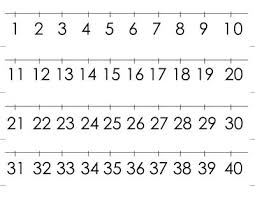# Thirteen 68474

I think the number. From its thirteen times, I'll subtract 97 and get the number 46. What number do I think?

x =  11

### Step-by-step explanation:Did you find an error or inaccuracy? Feel free to write us. Thank you!

Tips to related online calculators
Do you have a linear equation or system of equations and looking for its solution? Or do you have a quadratic equation?